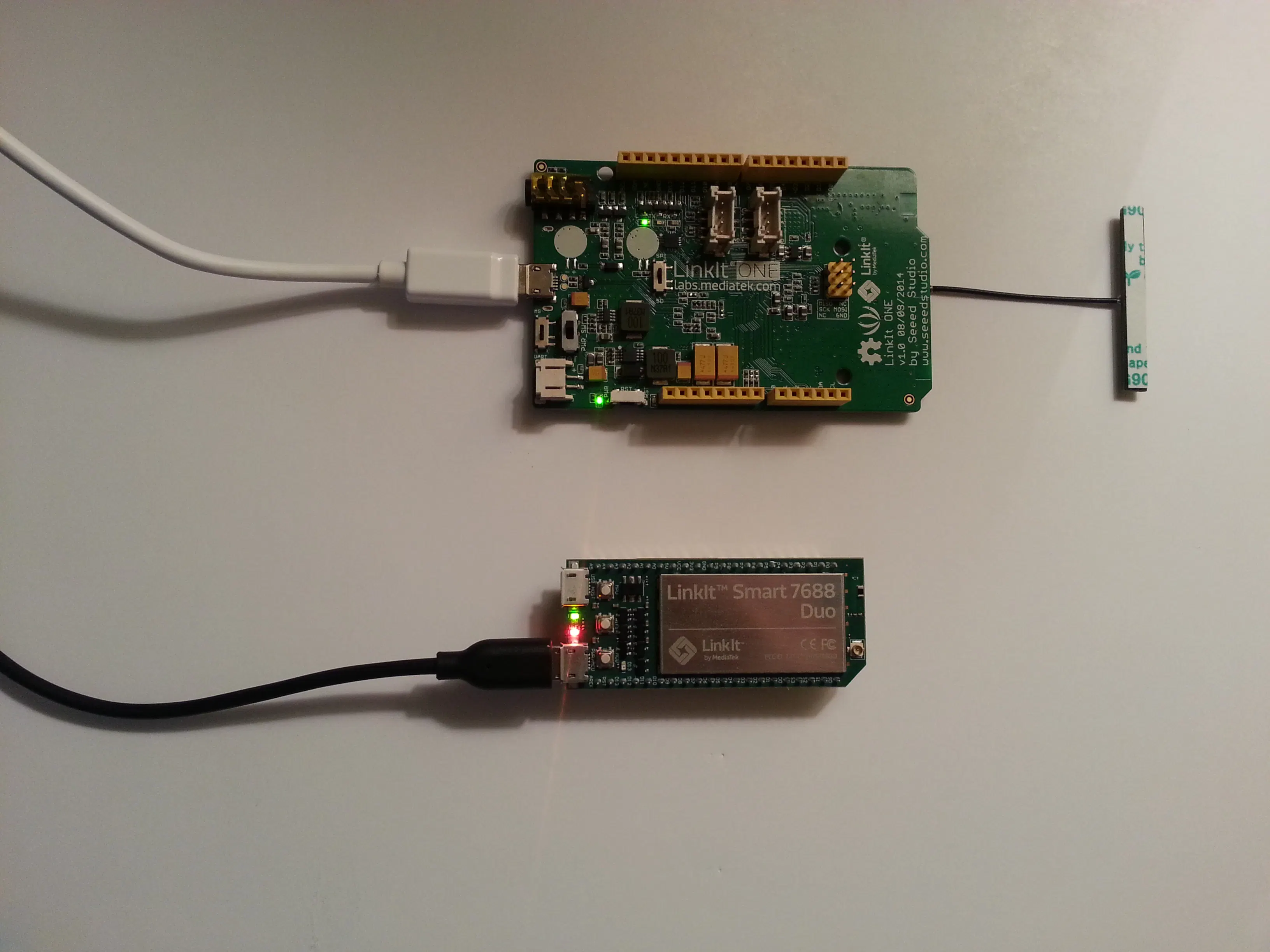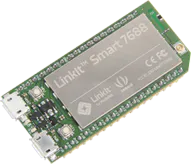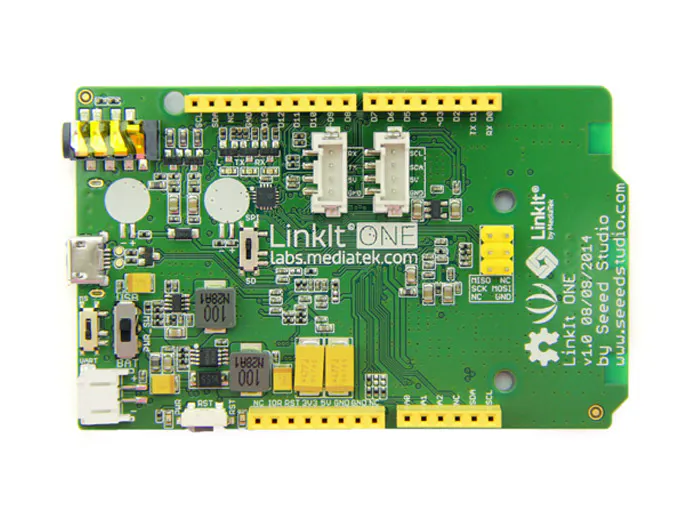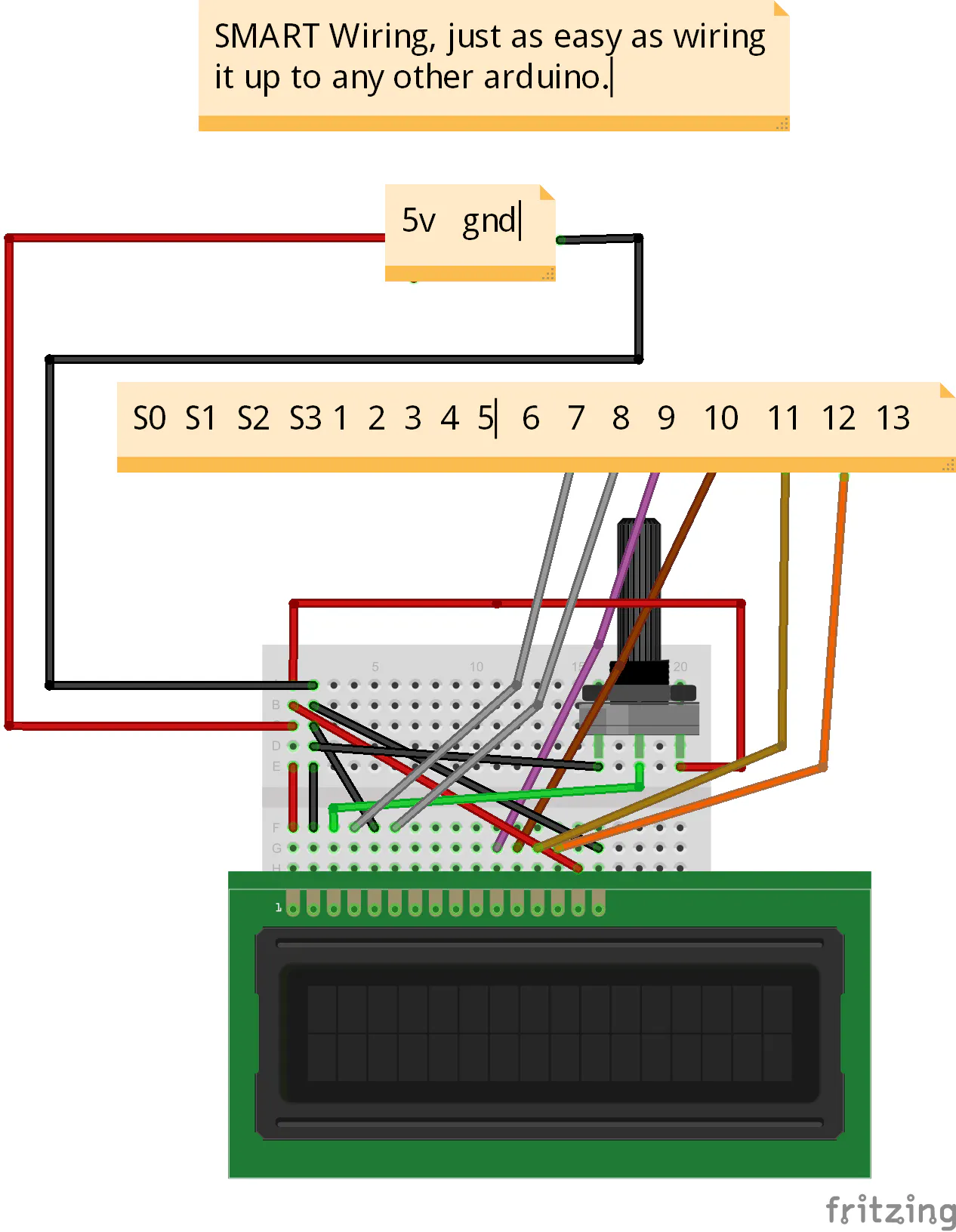Using two Linkit devices to monitor/track and notify movements and clicks within your home on a centralized router

IntermediateFull instructions provided7,486Things used in this project

Hardware components×1MediaTek Labs LinkIt ONE With WiFi antenna
×1
 Adafruit LCD Any non serial LCD will work, plug and play
×1
×1

Software apps and online services

 WinSCP
 PuTTyArduino IDE

Hand tools and fabrication machines

 Laptop Anything that preferably runs windows
 x2 Micro-Usb

Schematics

SMART (LCD) wiring

Just a visual of how to wire the SMART to the LCD, remember the notes are the SMART (pins). Sorry I don't know how to create boards on fritzing yetCode

Test1.py

Python
Use WinSCP to place this on the SMART for part one of the test for the COM between the ONE
```import SocketServer

IP=""
PORT=2011

class TCP(SocketServer.BaseRequestHandler):
def handle(self):
print "Got:\n{}".format(self.request.recv(1024).strip())
if __name__ == "__main__":
SocketServer.TCPServer((IP, PORT), TCP).serve_forever()
```

Test1(Arduino).ino

C/C++
Use this to flash the Linkit ONE to send a TCP packet(For testing) to the SMART
```#include <LTask.h>
#include <LWiFi.h>
#include <LWiFiClient.h>

#define ENTER LWIFI_OPEN
#define IP "192.168.100.1"
#define PORT 2011
#define LED 13

LWiFiClient client;
int number = 0;

void setup()
{
pinMode(LED, OUTPUT);
LWiFi.begin();
{
delay(500);
digitalWrite(LED, HIGH);
delay(500);
digitalWrite(LED, LOW);
}
digitalWrite(LED, LOW);

while (!(client.connect(IP, PORT)))
{
delay(200);
digitalWrite(LED, HIGH);
delay(200);
digitalWrite(LED, LOW);
}
digitalWrite(LED, HIGH);
}

void loop()
{
send("Hello From The ONE: "+String(number++));
delay(1000);
}

void send(String toSend)
{
while (!(client.connect(IP, PORT))) delay(50);
client.println(toSend);
client.stop();
}
```

Server(Duo).ino

C/C++
Open this up in arduino and flash it to the Atmel32u on the LinkIt SMART
```#include <LiquidCrystal.h>

LiquidCrystal lcd(7, 8, 9, 10, 11, 12);
String firstLine = "";
String secondLine = "";

void setup()
{
lcd.begin(16, 2);
lcd.print("Waiting for");
lcd.setCursor(0, 1);
lcd.print("Startup script");
Serial1.begin(57600);
delay(2);
delay(200);
lcd.clear();
}

void loop()
{
lcd.clear();
{
}
else if(rec.indexOf("%=new=%") != -1)
{
firstLine = rec.substring(0, rec.indexOf("%=new=%"));
secondLine = rec.substring(rec.indexOf("%=new=%")+7);
lcd.print(String(firstLine));
lcd.setCursor(0, 1);
lcd.print(String(secondLine));
}
else
{
lcd.print(String(rec));
}
}

{
while(!(Serial1.available()));
while (Serial1.available()) {
delay(3);
if (Serial1.available() > 0) {
}
}
return fin;
}
}
```

VIEW-SENSORS.html

HTML
Place this anywhere on your computer, edit the device and apikey then run
```<html>
<TITLE>SMART HOME</TITLE>
<style type="text/css">
body {
background-image:
}
</style>
<script type="text/javascript"
src="http://code.jquery.com/jquery-1.10.1.min.js"></script>
<body>
<code>
<p><center><big>ULTRASONIC SENSOR</big></center></p>
<div id="carriots-ultrasonic" style="max-width: 800px;margin:auto;"></div>
<p><center><big>POT SENSOR</big></center></p>
<div id="carriots-pot" style="max-width: 800px;margin:auto;"></div>
<p><center><big>SWITCH SENSOR</big></center></p>
<div id="carriots-sw" style="max-width: 800px;margin:auto;"></div>
</code>
<script src="https://www.carriots.com/js/graphs.js"></script>
<script type="text/javascript">
var carriots = [
{
"apikey": "APIKEYHERE",
"mime": "image/svg+xml",
"type": "line",
"axis[x]": "at",
"axis[y]": "distance",
"data": "activated",
"data": "distance",
"query[device]": "DEVICENAMEHERE",
"query[sort]": "at",
"query[order]": "asc",
"query[max]": "30",
"id": "carriots-ultrasonic"
},
{
"apikey": "APIKEYHERE",
"mime": "image/svg+xml",
"type": "line",
"axis[x]": "at",
"axis[y]": "turn",
"data": "activated",
"data": "turn",
"query[device]": "DEVICENAMEHERE",
"query[sort]": "at",
"query[order]": "asc",
"query[max]": "20",
"id": "carriots-pot"
},
{
"apikey": "APIKEYHERE",
"mime": "image/svg+xml",
"type": "line",
"axis[x]": "at",
"axis[y]": "onoff",
"data": "active",
"data": "switcher",
"query[device]": "DEVICENAMEHERE",
"query[sort]": "at",
"query[order]": "asc",
"query[max]": "10",
"id": "carriots-sw"
}
];
setTimeout(function(){
}, 21000);
</script>
</body>
</html>
```

porter.py

Python
Place this in the Python Carriot API folder, wherever you need on your PC not SMART
```from clicarriots.api import Stream
from datetime import datetime
from SocketServer import TCPServer, BaseRequestHandler
from socket import socket, AF_INET, SOCK_STREAM

IP = '192.168.100.1'
SERVIP=''
SERVPORT=2012
PORT = 2011
BUFFER_SIZE = 1024
CODE = "%=GET=%:{}".format(str(SERVPORT))
STOPCODE = "%=STOP=%"
APIKEY="PLACEAPIHERE"
DEVICE='DEVICENAMEHERE'

def sendCarriot(activated=0, distance=0,first="activated", second="distance"):
client_stream = Stream(APIKEY)
code, response = client_stream.send(DEVICE, {first:activated, second:distance}, datetime.now(),
'stream')
if not int(code) is 200:
print "Error sending %s" % str(response)
else:
print "Successfully sent to carriot!"

def sub(full, starter, ender):
return full[full.index(starter)+(len(starter)):full.index(ender)]

def find(full, finds):
if full.find(finds) != -1:
return True
return False

def exiter():
get = socket(AF_INET, SOCK_STREAM)
get.connect((IP, PORT))
get.send(STOPCODE)
get.close()

class TCP(BaseRequestHandler):
def handle(self):
self.data = self.request.recv(1024).strip()
if find(self.data, "%=EOF=%"):
if find(self.data, "%=ultra=%"):
val = sub(self.data, "%=ultra=%:", "%=EOF=%")
print "Ultrasonic:", val
sendCarriot(1, val)
elif find(self.data, "%=pot=%"):
val = sub(self.data, "%=pot=%:", "%=EOF=%")
print "Potentiometer: ", val
sendCarriot(1, val, "activated", "turn")
elif find(self.data, "%=sw=%"):
val = sub(self.data, "%=sw=%:", "%=EOF=%")
print "Switch Value: ", val
if val in "ON":
val = 100
else:
val = 0
sendCarriot(1, val, "active", "switcher")
def main():
get = socket(AF_INET, SOCK_STREAM)
get.connect((IP, PORT))
get.send(CODE)
get.close()
TCPServer((SERVIP, SERVPORT), TCP).serve_forever()
if __name__ == "__main__":
try:
main()
finally:
exiter()
```

Server.py

Python
Place this into the root folder on the LinkIt SMART, DONT MESS with the ipComputer
```from SocketServer import TCPServer, BaseRequestHandler
from socket import socket, AF_INET, SOCK_STREAM
from serial import Serial

IP = ""
PORT = 2011
global mt, ipComputer #don't mess I will handle it
mt = None
BUFFER_SIZE = 1024

def sender(data):
send = socket(AF_INET, SOCK_STREAM)
send.connect(ipComputer)
send.send(data)
send.close()

class TCP(BaseRequestHandler):
def handle(self):
global ipComputer
mtSend = recv
self.data = self.request.recv(1024).strip()
# print "Got:\n{}".format(self.data)
if self.data.find("%=connect=%") != -1:
recv = "Got Connected to Device"
mtSend = "Got Connected %=new=%to Device"
elif (self.data.find("%=ultra=%") != -1) and (self.data.find("%=EOF=%") != -1):
recv = self.data[self.data.index("%=ultra=%:")+10:self.data.index("%=EOF=%")]
datas = str(self.data)
mtSend = "Ultrasonic:%=new=%"+recv+" inches"
recv += " Ultrasonic (inches)"
if ipComputer != None:
elif self.data.find("%=GET=%") != -1:
recv = "Porting data to"
recv += " %s Server" % str(ipComputer)
elif self.data.find("%=STOP=%") != -1:
recv = "Stopping to send"
ipComputer = None
mtSend = recv+"%=new=%to Server"
recv += " %s to Server" % str(ipComputer)
elif (self.data.find("%=pot=%") != -1) and (self.data.find("%=EOF=%")):
recv = self.data[self.data.index("%=pot=%:")+8:self.data.index("%=EOF=%")]
datas = str(self.data)
mtSend = "Pot Value: %=new=%("+recv+"/1023)"
recv += "/1023 (Pot)"
if ipComputer != None:
elif (self.data.find("%=sw=%") != -1) and (self.data.find("%=EOF=%")):
recv = self.data[self.data.index("%=sw=%:")+7:self.data.index("%=EOF=%")]
datas = str(self.data)
mtSend = "Switch Value: %=new=%"+recv
recv += " (Switch)"
if ipComputer != None:
elif len(self.data) > 3:
recv += str(self.data)
mtSend += "%=new=%"+(str(self.data))
else:
recv = ""
mtSend = recv
if ipComputer is None:
print recv
mt.write(str(mtSend))

if __name__ == "__main__":
global ipComputer, datas
datas = ["0", "0", "OFF"]
ipComputer = None
mt = Serial("/dev/ttyS0", 57600)
TCPServer((IP, PORT), TCP).serve_forever()
```

ONE.ino

C/C++
Flash this to your LinkIt one and just properly wire it, no code modifications should be required
```#include <LTask.h>
#include <LWiFi.h>
#include <LWiFiClient.h>

#define ENTER LWIFI_OPEN
#define IP "192.168.100.1"
#define PORT 2011
#define LED 13
#define trigPin 11
#define echoPin 12
#define potPin A0
#define switchPin 2

LWiFiClient client;
int number = 0;
long last = 0;
int lastPot = 0;
boolean lastSwitch = false;

void setup()
{
pinMode(trigPin, OUTPUT);
pinMode(echoPin, INPUT);
pinMode(potPin, INPUT);
pinMode(LED, OUTPUT);
pinMode(switchPin, INPUT);
LWiFi.begin();
{
delay(500);
digitalWrite(LED, HIGH);
delay(500);
digitalWrite(LED, LOW);
}
digitalWrite(13, LOW);

while (!(client.connect(IP, PORT)))
{
delay(200);
digitalWrite(LED, HIGH);
delay(200);
digitalWrite(LED, LOW);
}
client.stop();
send("%=connect=%");
digitalWrite(LED, HIGH);
last = ultraSonic();
lastPot = pot();
lastSwitch = sw();
}

void loop()
{
long distance = ultraSonic();
if(((distance-last)< -4) ||((distance-last)> 4))
{
send("%=ultra=%:"+String(distance)+"%=EOF=%");
delay(500);
}
int potVal = pot();
while(((potVal-lastPot)< -2) ||((potVal-lastPot)> 2))
{
send("%=pot=%:"+String(potVal)+"%=EOF=%");
lastPot = potVal;
delay(100);
potVal = pot();
}
digitalWrite(LED, sw());
boolean switchVal = sw();
if(switchVal != lastSwitch)
{
if(switchVal)
{
send("%=sw=%:ON%=EOF=%");
}
else
{
send("%=sw=%:OFF%=EOF=%");
}
lastSwitch = switchVal;
}
last = distance;
delay(500);
}

void send(String toSend)
{
while (!(client.connect(IP, PORT)))
delay(50);
client.println(toSend);
client.stop();
}

long ultraSonic()
{
long duration;
digitalWrite(trigPin, LOW);
delayMicroseconds(2);
digitalWrite(trigPin, HIGH);
delayMicroseconds(10);
digitalWrite(trigPin, LOW);
duration = pulseIn(echoPin, HIGH);
return ((duration/2) / 29.1);
}

int pot()
{
}

int sw()
{